GFG App
Open AppBrowser
Continue

Just as static electric charges create an electric field, moving charges create a magnetic field. Therefore, a moving charge creates both an electric and a magnetic field around itself and exerts a force known as the electromagnetic force, which is one of the fundamental forces of nature. This force cannot be directly experienced by humans, but we can observe its two components in real-life situations. For instance, rubbing a comb with woolen cloth can attract small pieces of paper and is an example of electric force. Magnets, on the other hand, exert magnetic fields. Moreover, if iron filings are placed on paper with a magnet placed under the paper, the iron filings align in the direction of the magnetic force, and a pattern of magnetic field lines emerges.

## What is Magnetic Field?

Magnetic field is the region around a magnet or current-carrying conductor around which the force of magnetism can be observed. It is a vector quantity and is generated by moving charges. Magnetic forces and Electric forces are part of one of the four fundamental forces of nature. Electric and Magnetic forces interplay with each other to form electromagnetic forces which are an essential part of our modern engineering infrastructure and development.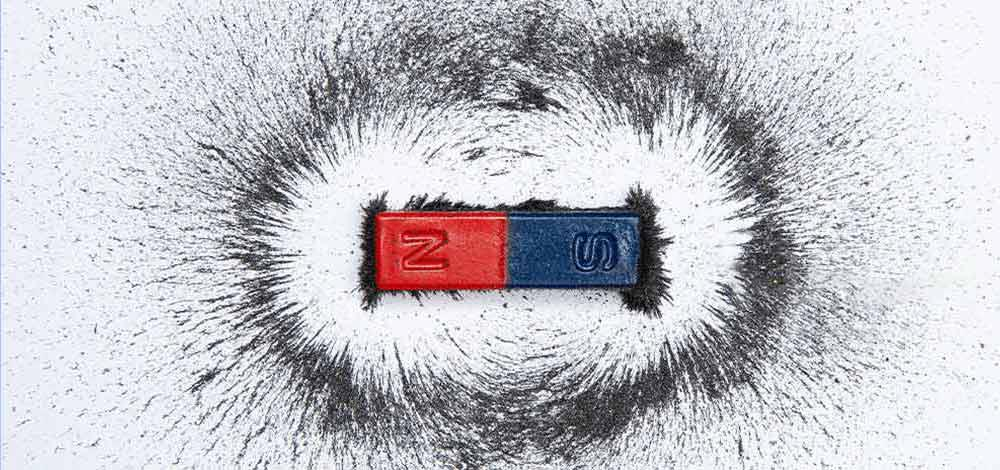## History of Magnetic Field

The research about magnetic fields was first observed in the 13th century when a french scholar Petrus Peregrinus de Maricourt used iron needles to map out the magnetic field lines around a sphere magnet. The points where these lines crossed, he names them poles. He stated that magnets have poles – North and South. Centuries later William Gilbert claimed in his experiments that the earth is a magnet. After that observation, during the 18th and 19th centuries, several theorists and researchers came up with theories around attraction and repulsion in magnetic poles. English scientist Faraday discovered that changing magnetic fields produce electricity and gave the theory of electromagnetic induction. Later, James Maxwell published theories on electricity and magnetic field intensities and their interplay. They came to be known as Maxwell’s Equation and are an integral part of modern engineering.

## Illustration of Magnetic Field

A Magnet is a material that has the ability to produce a magnetic field which creates magnetic field lines, the field force is able to attract and repel other ferromagnetic materials like iron, nickel, etc. The magnetic field can be illustrated using two possible ways – Magnetic Field Vector or Magnetic Field Lines.

## Magnetic Vector Field

The magnetic field is a vector quantity (it has both magnitude and direction), and the direction of the magnetic field is from the north pole to the South Pole. It is a vector field, mathematically which means it is a set of many vectors which points in different directions. The figure below illustrates a magnetic field using a magnetic field vector. The length of these vectors determines the strength of the field at that particular point.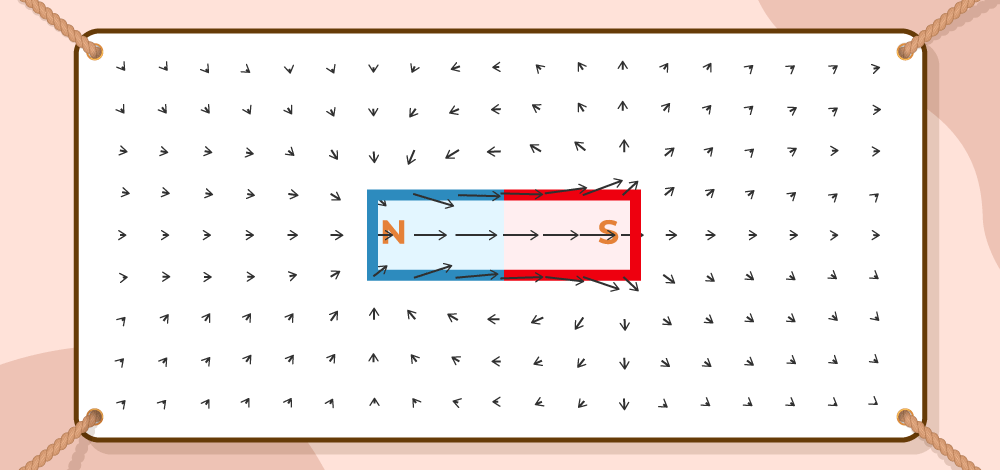## Magnetic Field Lines

Another representation of the magnetic field can be done using the magnetic field lines which are shown in the figure below. The strength of the magnetic field is shown here by the closeness of magnetic field lines. For example, magnetic field is stronger at the poles because the lines are more crowded in that area.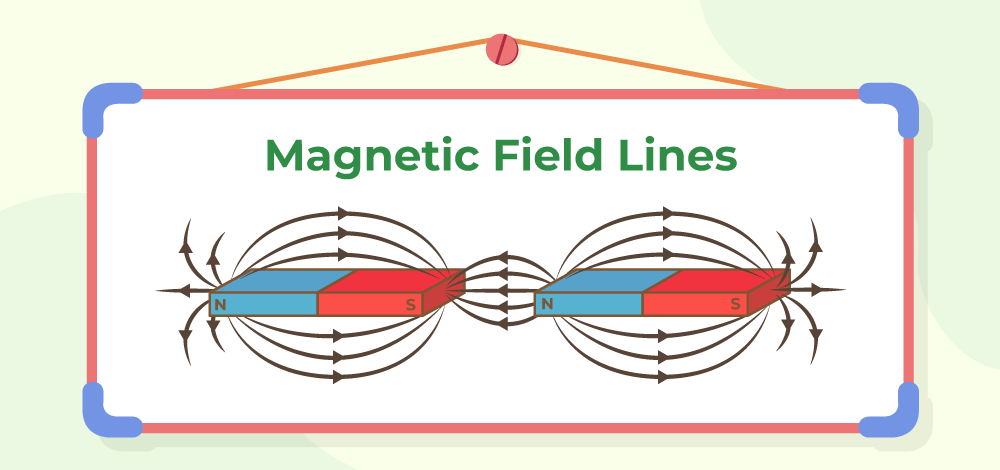### Properties of Magnetic Field Lines

• Magnetic Field lines never cross each other.
• Magnetic Field lines always form closed loops, outside of the magnet they travel from the North to the South pole, and inside the magnet, they travel from the south to the north pole.
• The density of the magnetic field lines indicates the strength of the magnetic field in that area.

## Magnetic Field Intensity

Magnetic field Intensity denotes the strength of the magnetic field. It is represented by H and is a vector quantity. It is defined as the magnetomotive force(MMF) needed to create a flux density B inside a material per unit length of that material. The unit of Magnetic Field intensity is Ampere/Meter and the dimensional formula for the same is given by [M1T-2I-1]. SI unit of Magnetic Field Intensity is Tesla.  A magnetic field of strength One Tesla can also be thought of as the magnetic field producing a force of 1 Newton per ampere of current in each meter of conductor.here, B is the magnetic flux density, M is magnetization and μ is magnetic permeability.

### Magnetic Effect of Electric Current

When current is passed through a magnetic material, a magnetic field is produced, if a needle is placed near the material, providing the electric charge (moving charges), the needle will deflect. The area to the point where the force is exerted by the magnetic material is called a magnetic field.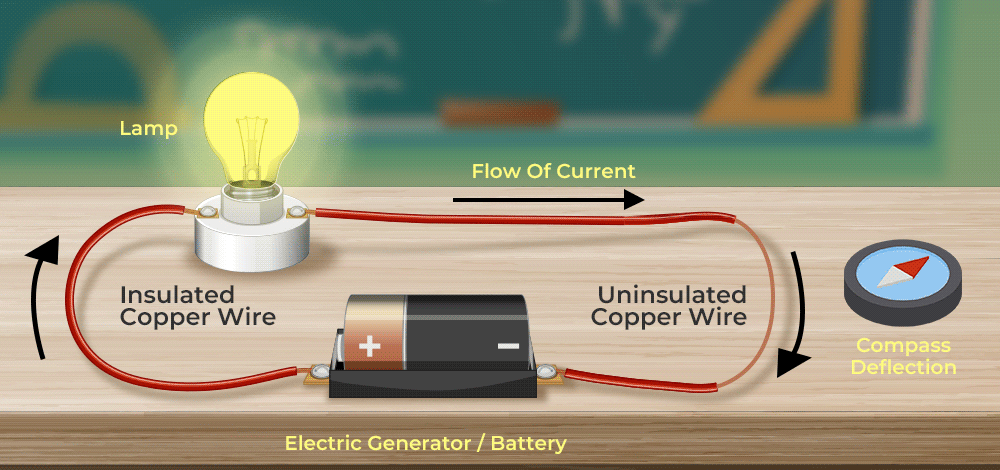Properties of Magnetic Field:

• The strength of the magnetic field is directly proportional to the density of the field lines produced by them.
• Magnetic field lines never cross each other.
• Magnetic fields will always have closed and continuous loops.

## Magnetic Field lines due to Current Carrying Straight Conductor

The magnetic field lines produced by a straight current-carrying conductor is in the form of concentric circles, depending upon the direction of the current, magnetic field lines also change their directions. The direction of the magnetic field lines can be identified by Right-hand Thumb Rule or Maxwell’s corkscrew rule.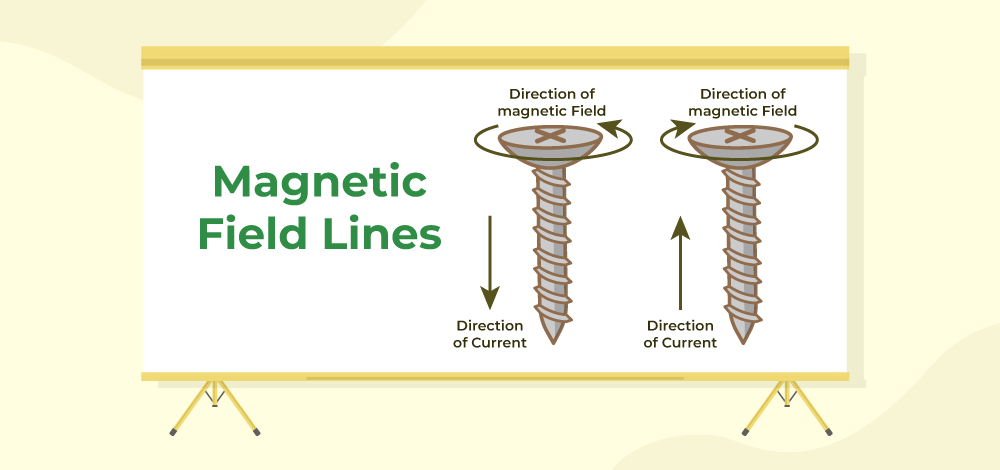### Right-Hand Thumb Rule

According to Right- Hand Thumb Rule, If the right hand’s thumb is placed in the direction of the moving current, then the direction of the fingers shows the direction of magnetic field lines, Similarly, if the thumb is placed in the direction of the magnetic field lines, then the direction of current is shown by the curled fingers’ direction.

### Maxwell’s Corkscrew Rule

According to Maxwell’s Corkscrew rule, A screw can tell the direction of the magnetic field lines. If the screw is moved forward, the direction of rotation shows the direction of magnetic field lines while the direction of the movement of the screw shows the direction of current and Vice-Versa.

## Magnetic Field lines due to Current through a Circular Loop

The magnetic field lines will create the same pattern as they created in the case of a straight current-carrying conductor, That means, the field lines will be concentric in nature, but based on the direction of the current, the direction of field lines will change as well. For example, in a circular loop, imagine two places, one where the current goes upward and one where the current goes downward, according to the right-hand thumb rule, in the first case, the field lines must be anticlockwise in nature, and in the second case, the field lines must be clockwise in nature.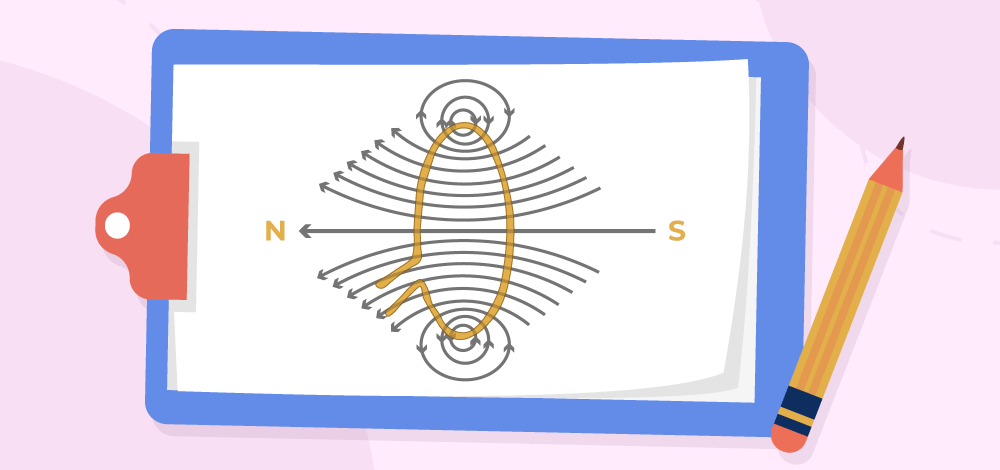## Magnetic Field lines due to Current in Solenoid

A solenoid is a helical coil which upon giving current becomes an electromagnet and forms North and South Pole and due to moving charges, it creates Magnetic field lines. The field lines created by the solenoid look similar to the field lines created by a simple Bar magnet.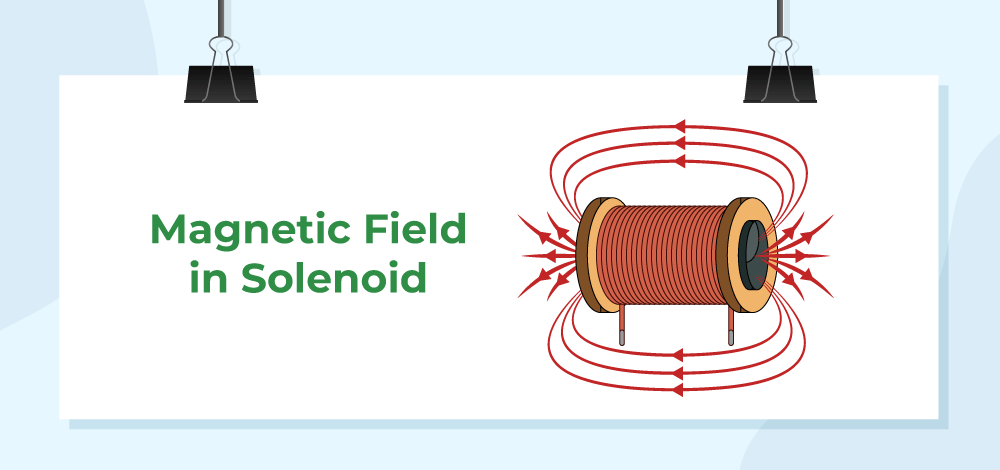## Electromagnet

An electromagnet is a type of magnet formed due to the current moving and passing through it, it is a type of temporary magnet and is mostly seen as a coil wrapped over a soft iron core (for example, solenoid).

### Properties of electromagnet

• The electromagnets are not permanent magnets, they are temporary in nature.
• The strength of the magnetic force can be altered by varying currents.
• Electromagnets are made with soft iron cores.

Note: When current is applied on a ferromagnetic material to produce magnetic field lines, the magnetic force is dependent on the number of magnetic dipole aligned, The amount of current applied is directly proportional to the number of dipoles alignment and the amount of magnetic force can be altered. However, it can only be done up to some point, till the point where saturation reaches and no dipoles can further be available for alignment.

## Force on a current-carrying conductor placed in a Magnetic Field

Imagine a wire (conductor) is placed under a magnet and current is passed through the wire, due to the moving electric charges in a magnetic field, a force is applied to the conductor that moves it in a certain direction, this phenomenon was given by Michael Faraday. The direction of the force on the conductor is determined by Fleming’s left-hand rule.

### Fleming’s Left-hand Rule

According to Fleming’s left-hand rule, the direction of force can be determined with the help of left hand’s thumb, index finger, and middle finger. The thumb represents the Force, The index finger shows the direction of the magnetic field lines and the middle finger shows the direction of the current. Therefore, if the middle finger and index finger are kept in the direction of current and magnetic field lines respectively, the thumb will represent the direction of force produced on current carrying conductor.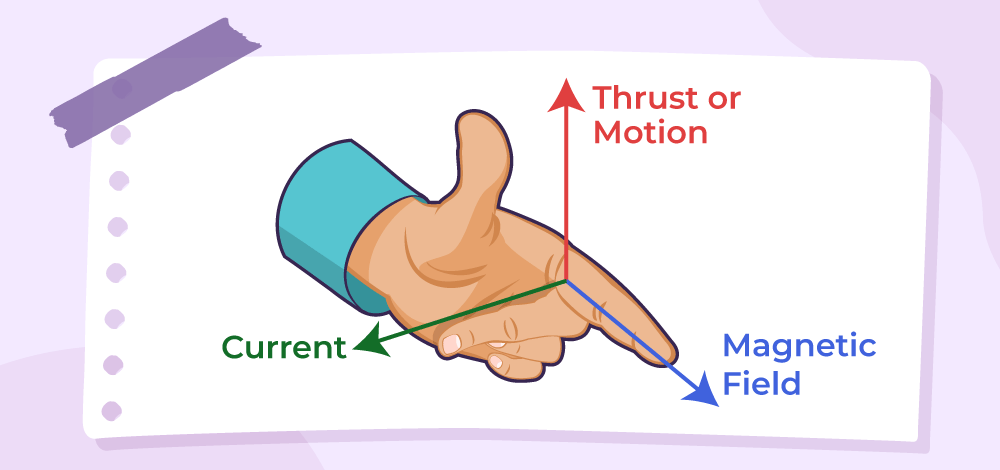## Sample Questions on Magnetic Field

Question 1: What are the applications of Fleming’s left-hand rule?

Fleming’s left-hand rule is applied in Electric motors (DC motors, induction motors, etc). The basic concept is that the induced current produces force on the conductor which helps in creating motion.

Question 2: What is the difference between a Permanent magnet and an electromagnet?

A Permanent magnet is made up of Hard material, The magnetic field is produced due to magnetization and once the magnetization is lost, the magnet ceases to show magnetic properties since the magnetic dipoles cannot be changed further.

An electromagnet is made up of Soft material, The magnetic field is produced by applying current through it. Once the current stops, the magnetism stops but again develops after applying current again, The dipoles aligned in an electromagnet can be altered again and again.

Question 3: Write down the Properties of Magnetic field lines.

Properties of magnetic field lines

• The magnetic field lines decide the strength of the magnetic field, denser the field lines, stronger the magnetic field.
• They always form closed and continuous loops.
• Magnetic field lines never cross each other, since they do not have two directions.

## FAQs on Magnetic Field

### Question 1: What is a magnetic field?

Magnetic Field is a vector field in a region around the magnet or moving charges where the force of magnetism acts.

### Question 2: What causes earth’s magnetic field?

Magnetic field of earth is generated inside the core of earth due to flow of liquid iron at the centre. Due to these flows, electric current is generated and thus a changing magnetic field is generated which in turn generates more current. This cycle continues and generates a magnetic field around earth.

### Question 3: Why don’t two magnetic field lines intersect each other?

Since magnetic field is a vector field, the lines denote the direction of magnetic field. If two magnetic field lines intersect each other, there will be two directions of the magnetic field at a single point which is not possible. Thus, magnetic field lines do not intersect each other.

### Question 4: Why are magnetic flux lines important?

Magnetic flux lines are the lines of magnetic field, the tangent at any point to these lines denote the direction of the magnetic field at that particular point. Their density gives us the idea about the strength of the magnetic field in a region. The density of these lines are higher at the poles, that is why poles have very strong magnetic field strength in their vicinity.

### Question 5: Why do magnetic field lines vary in density?

Magnetic field lines can vary in density because the field lines start near the magnet, and then they go further away and as they go further away from the magnet, the effect of magnetic force gradually decreases and stops after a point, since the force is decreasing, the density of the magnetic field lines also tend to decrease.

### Question 6: List two methods for creating a magnetic field.

Magnetic Field can be generated by following two methods:

1. Magnet Bar, horse-shoe magnet or a round magnet.
2. Moving current carrying condutor, solenoid etc.